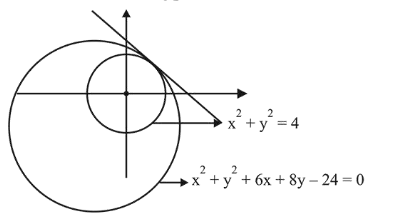# The common tangent to the circlesQuestion:

The common tangent to the circles $x^{2}+y^{2}=4$ and $x^{2}+y^{2}+6 x+8 y-24=0$ also passes through the point:

1. (1) $(4,-2)$

2. (2) $(-6,4)$

3. (3) $(6,-2)$

4. (4) $(-4,6)$

Correct Option: , 3

Solution:

By the diagram, $d_{c_{1} c_{2}}=\left|r_{1}-r_{2}\right|$Equation of common tangent is,

$\mathrm{S}_{1}-\mathrm{S}_{2}=0$

$6 x+8 y-20=0 \Rightarrow 3 x+4 y-10=0$

Hence $(6,-2)$ lies on it.